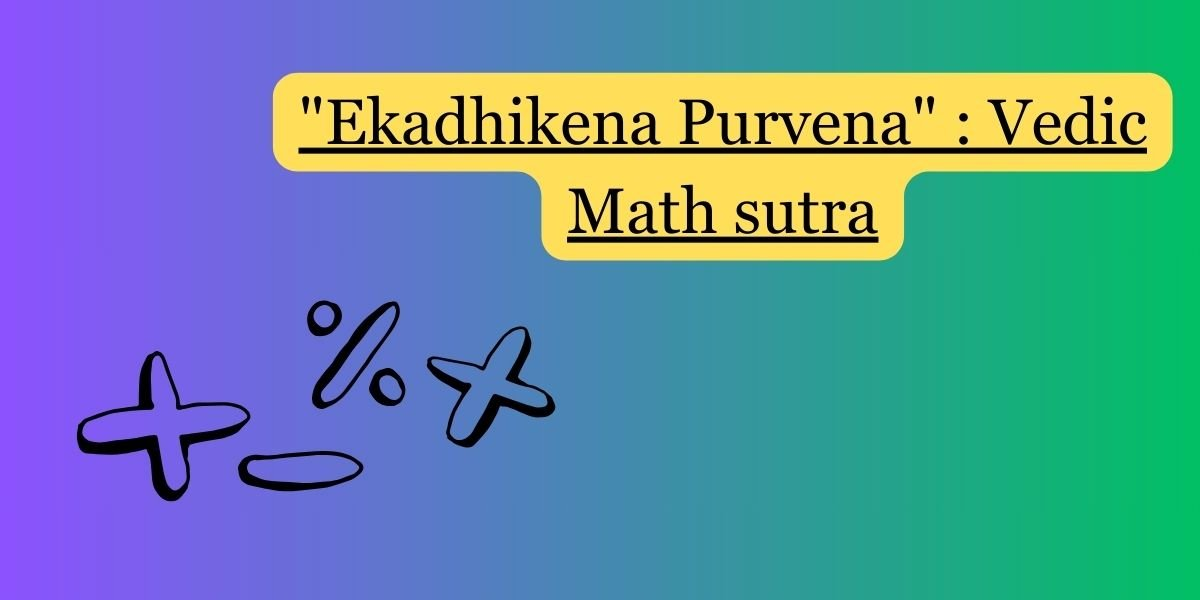“Ekadhikena Purvena” is one of the Vedic Math sutras, which translates to “By One More than the Previous One” in English. This sutra is used to simplify multiplication when the multiplier is one more than the base. The sutra is particularly helpful when dealing with numbers that are close to a power of 10.

The general form of the sutra is as follows:

``Ekadhikena Purvena: ab×(a+1)=a(a+1) concatenate (10−a)``

Here, ab is a two-digit number, and a is the digit in the tens place.

Let’s take an example to illustrate:

43×44

Here, a = 4, and b = 3 Applying the sutra:

``43×44=4(4+1) concatenate (10−4)=4×5 concatenate 6=1892``

So, according to the Ekadhikena Purvena sutra, 43 multiplied by 44 is 1892.

This sutra is a mental math technique that can be useful for quick calculations, especially when you’re dealing with numbers that are close to each other or close to multiples of 10.